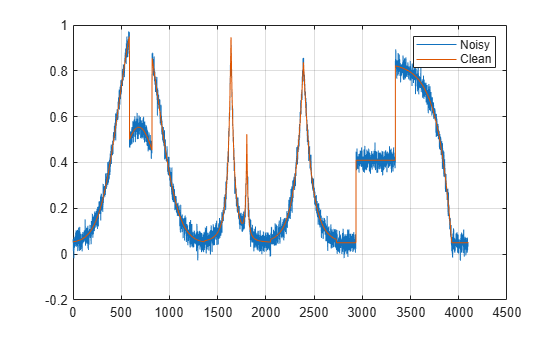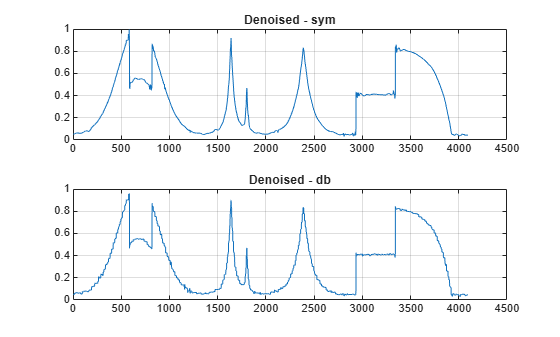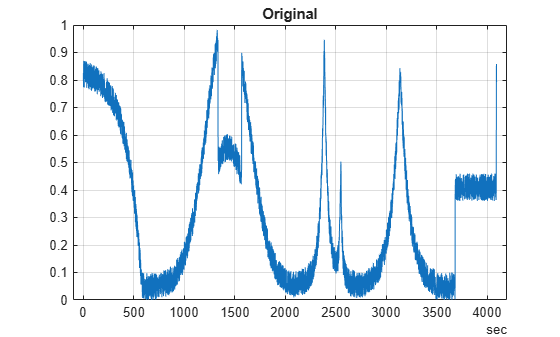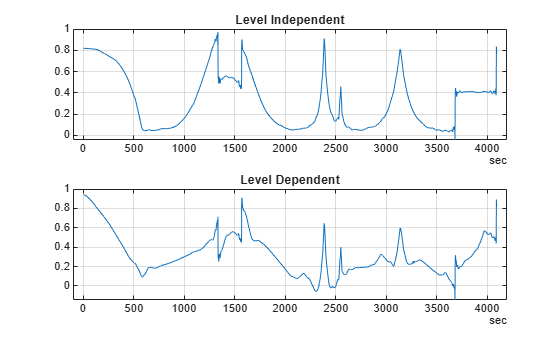# wdenoise

Wavelet signal denoising

## Syntax

``XDEN = wdenoise(X)``
``XDEN = wdenoise(X,LEVEL)``
``XDEN = wdenoise(___,Name,Value)``
``[XDEN,DENOISEDCFS] = wdenoise(___)``
``[XDEN,DENOISEDCFS,ORIGCFS] = wdenoise(___)``

## Description

example

````XDEN = wdenoise(X)` denoises the data in `X` using an empirical Bayesian method with a Cauchy prior. By default, the `sym4` wavelet is used with a posterior median threshold rule. Denoising is down to the minimum of `floor(log2N)` and `wmaxlev(N,'sym4')` where N is the number of samples in the data. (For more information, see `wmaxlev`.) `X` is a real-valued vector, matrix, or timetable. If `X` is a matrix, `wdenoise` denoises each column of `X`. If `X` is a timetable, `wdenoise` must contain real-valued vectors in separate variables, or one real-valued matrix of data.`X` is assumed to be uniformly sampled.If `X` is a timetable and the timestamps are not linearly spaced, `wdenoise` issues a warning. ```
````XDEN = wdenoise(X,LEVEL)` denoises `X` down to `LEVEL`. `LEVEL` is a positive integer less than or equal to `floor(log2N)` where N is the number of samples in the data. If unspecified, `LEVEL` defaults to the minimum of `floor(log2N)` and `wmaxlev(N,'sym4')`.```

example

````XDEN = wdenoise(___,Name,Value)` specifies options using name-value pair arguments in addition to any of the input arguments in previous syntaxes.```
````[XDEN,DENOISEDCFS] = wdenoise(___)` returns the denoised wavelet and scaling coefficients in the cell array `DENOISEDCFS`. The elements of `DENOISEDCFS` are in order of decreasing resolution. The final element of `DENOISEDCFS` contains the approximation (scaling) coefficients.```
````[XDEN,DENOISEDCFS,ORIGCFS] = wdenoise(___)` returns the original wavelet and scaling coefficients in the cell array `ORIGCFS`. The elements of `ORIGCFS` are in order of decreasing resolution. The final element of `ORIGCFS` contains the approximation (scaling) coefficients.```

## Examples

collapse all

Obtain the denoised version of a noisy signal using default values.

```load noisdopp xden = wdenoise(noisdopp);```

Plot the original and denoised signals.

```plot([noisdopp' xden']) legend('Original Signal','Denoised Signal')```Denoise a timetable of noisy data down to level 5 using block thresholding.

`load wnoisydata`

Denoise the data down to level 5 using block thresholding by setting the name-value pair `'DenoisingMethod','BlockJS'`.

`xden = wdenoise(wnoisydata,5,'DenoisingMethod','BlockJS');`

Plot the original data and the denoised data.

```h1 = plot(wnoisydata.t,[wnoisydata.noisydata(:,1) xden.noisydata(:,1)]); h1(2).LineWidth = 2; legend('Original','Denoised')```Denoise a signal in different ways and compare results.

Load a datafile that contains clean and noisy versions of a signal. Plot the signals.

```load fdata.mat plot(fNoisy,'r-') hold on plot(fClean,'b-') grid on legend('Noisy','Clean');```Denoise the signal using the `sym4` and `db1` wavelets, with a nine-level wavelet decomposition. Plot the results.

```cleansym = wdenoise(fNoisy,9,'Wavelet','sym4'); cleandb = wdenoise(fNoisy,9,'Wavelet','db1'); figure plot(cleansym) title('Denoised - sym') grid on``````figure plot(cleandb) title('Denoised - db') grid on```Compute the SNR of each denoised signal. Confirm that using the `sym4` wavelet produces a better result.

`snrsym = -20*log10(norm(abs(fClean-cleansym))/norm(fClean))`
```snrsym = 35.9623 ```
`snrdb = -20*log10(norm(abs(fClean-cleandb))/norm(fClean))`
```snrdb = 32.2672 ```

Load in a file which contains noisy data of 100 time series. Every time series is a noisy version of `fClean`. Denoise the time series twice, estimating the noise variance differently in each case.

```load fdataTS.mat cleanTSld = wdenoise(fdataTS,9,'NoiseEstimate','LevelDependent'); cleanTSli = wdenoise(fdataTS,9,'NoiseEstimate','LevelIndependent');```

Compare one of the noisy time series with its two denoised versions.

```figure plot(fdataTS.Time,fdataTS.fTS15) title('Original') grid on``````figure plot(cleanTSli.Time,cleanTSli.fTS15) title('Level Independent') grid on``````figure plot(cleanTSld.Time,cleanTSld.fTS15) title('Level Dependent') grid on```## Input Arguments

collapse all

Input data, specified as a matrix, vector, or timetable of real values. If `X` is a vector, it must have at least two samples. If `X` is a matrix or timetable, it must have at least two rows.

Data Types: `double`

Level of wavelet decomposition, specified as a positive integer. `LEVEL` is a positive integer less than or equal to `floor(log2N)` where N is the number of samples in the data.

• If unspecified, `LEVEL` defaults to the minimum of `floor(log2N)` and `wmaxlev(N,'sym4')`.

• For James-Stein block thresholding, `'BlockJS'`, there must be `floor(log2N)` coefficients at the coarsest resolution level, `LEVEL`.

Data Types: `double`

### Name-Value Arguments

Specify optional comma-separated pairs of `Name,Value` arguments. `Name` is the argument name and `Value` is the corresponding value. `Name` must appear inside quotes. You can specify several name and value pair arguments in any order as `Name1,Value1,...,NameN,ValueN`.

Example: `'Wavelet','db6','DenoisingMethod','Bayes'` denoises using the Daubechies `db6` wavelet and the empirical Bayesian method.

Name of wavelet, specified as a character array, to use for denoising. The wavelet must be orthogonal or biorthogonal. Orthogonal and biorthogonal wavelets are designated as type 1 and type 2 wavelets respectively in the wavelet manager, `wavemngr`.

• Valid built-in orthogonal wavelet families begin with `haar`, `dbN`, `fkN`, `coifN`, or `symN` where `N` is the number of vanishing moments for all families except `fk`. For `fk`, `N` is the number of filter coefficients.

• Valid biorthogonal wavelet families begin with `'biorNr.Nd'` or `'rbioNd.Nr'`, where `Nr` and `Nd` are the number of vanishing moments in the reconstruction (synthesis) and decomposition (analysis) wavelet.

Determine valid values for the vanishing moments by using `waveinfo` with the wavelet family short name. For example, enter `waveinfo('db')` or `waveinfo('bior')`. Use `wavemngr('type',WNAME)` to determine if a wavelet is orthogonal (returns 1) or biorthogonal (returns 2).

Denoising method used to determine the denoising thresholds for the data `X`.

• `Bayes` — Empirical Bayes

This method uses a threshold rule based on assuming measurements have independent prior distributions given by a mixture model. Because measurements are used to estimate the weight in the mixture model, the method tends to work better with more samples. By default, the posterior median rule is used to measure risk .

• `BlockJS` — Block James-Stein

This method is based on determining an `optimal block size and threshold. The resulting block thresholding estimator yields simultaneously optimal global and local adaptivity .

• `FDR` — False Discovery Rate

This method uses a threshold rule based on controlling the expected ratio of false positive detections to all positive detections. The `FDR` method works best with sparse data. Choosing a ratio, or Q-value, less than `1/2` yields an asymptotically minimax estimator .

• `Minimax` — Minimax Estimation

This method uses a fixed threshold chosen to yield minimax performance for mean square error against an ideal procedure. The minimax principle is used in statistics to design estimators. See `thselect` for more information.

• `SURE` — Stein's Unbiased Risk Estimate

This method uses a threshold selection rule based on Stein’s Unbiased Estimate of Risk (quadratic loss function). One gets an estimate of the risk for a particular threshold value (t). Minimizing the risks in (t) gives a selection of the threshold value.

• `UniversalThreshold` - Universal Threshold $\sqrt{2\mathrm{ln}\left(\text{length}\left(x\right)\right)}.$

This method uses a fixed-form threshold yielding minimax performance multiplied by a small factor proportional to `log(length(X))`.

Note

For `'FDR'`, there is an optional argument for the Q-value, which is the proportion of false positives. Q is a real-valued scalar between `0` and `1/2`, `0 < Q <= 1/2`. To specify `'FDR'` with a Q-value, use a cell array where the second element is the Q-value. For example, `'DenoisingMethod',{'FDR',0.01}`. If unspecified, Q defaults to `0.05`.

Threshold rule, specified as a character array, to use to shrink the wavelet coefficients. `'ThresholdRule'` is valid for all denoising methods, but the valid options and defaults depend on the denoising method. Rules possible for different denoising methods are specified as follows:

• `'BlockJS'` — The only supported option is `'James-Stein'`. You do not need to specify `ThresholdRule` for `'BlockJS'`.

• `'SURE'`, `'Minimax'`, `'UniversalThreshold'` — Valid options are `'Soft'` or `'Hard'`. The default is `'Soft'`.

• `'Bayes'` — Valid options are `'Median'`, `'Mean'`, `'Soft'`, or `'Hard'`. The default is `'Median'`.

• `'FDR'` — The only supported option is `'Hard'`. You do not need to define `ThresholdRule` for `'FDR'`

Method of estimating variance of noise in the data.

• `'LevelIndependent'` — Estimate the variance of the noise based on the finest-scale (highest-resolution) wavelet coefficients.

• `'LevelDependent'` — Estimate the variance of the noise based on the wavelet coefficients at each resolution level.

Specifying `NoiseEstimate` with the `'BlockJS'` denoising method has no effect. The block James-Stein estimator always uses a `'LevelIndependent'` noise estimate.

## Output Arguments

collapse all

Denoised vector, matrix, or timetable version of `X`. For timetable input, `XDEN` has the same variable names and timestamps as the original timetable.

Data Types: `double`

Denoised wavelet and scaling coefficients of the denoised data `XDEN`, returned in a cell array. The elements of `DENOISEDCFS` are in order of decreasing resolution. The final element of `DENOISEDCFS` contains the approximation (scaling) coefficients.

Data Types: `double`

Original wavelet and scaling coefficients of the data `X`, returned in a cell array. The elements of `ORIGCFS` are in order of decreasing resolution. The final element of `ORIGCFS` contains the approximation (scaling) coefficients.

Data Types: `double`

## Algorithms

The most general model for the noisy signal has the following form:

`$s\left(n\right)=f\left(n\right)+\sigma e\left(n\right),$`

where time n is equally spaced. In the simplest model, suppose that e(n) is a Gaussian white noise N(0,1), and the noise level σ is equal to 1. The denoising objective is to suppress the noise part of the signal s and to recover f.

The denoising procedure has three steps:

1. Decomposition — Choose a wavelet, and choose a level `N`. Compute the wavelet decomposition of the signal s at level `N`.

2. Detail coefficients thresholding — For each level from 1 to `N`, select a threshold and apply soft thresholding to the detail coefficients.

3. Reconstruction — Compute wavelet reconstruction based on the original approximation coefficients of level `N` and the modified detail coefficients of levels from 1 to `N`.

More details about threshold selection rules are in Wavelet Denoising and Nonparametric Function Estimation and in the help of the `thselect` function.

 Abramovich, F., Y. Benjamini, D. L. Donoho, and I. M. Johnstone. “Adapting to Unknown Sparsity by Controlling the False Discovery Rate.” Annals of Statistics, Vol. 34, Number 2, pp. 584–653, 2006.

 Antoniadis, A., and G. Oppenheim, eds. Wavelets and Statistics. Lecture Notes in Statistics. New York: Springer Verlag, 1995.

 Cai, T. T. “On Block Thresholding in Wavelet Regression: Adaptivity, Block size, and Threshold Level.” Statistica Sinica, Vol. 12, pp. 1241–1273, 2002.

 Donoho, D. L. “Progress in Wavelet Analysis and WVD: A Ten Minute Tour.” Progress in Wavelet Analysis and Applications (Y. Meyer, and S. Roques, eds.). Gif-sur-Yvette: Editions Frontières, 1993.

 Donoho, D. L., I. M. Johnstone. “Ideal Spatial Adaptation by Wavelet Shrinkage.” Biometrika, Vol. 81, pp. 425–455, 1994.

 Donoho, D. L. “De-noising by Soft-Thresholding.” IEEE Transactions on Information Theory, Vol. 42, Number 3, pp. 613–627, 1995.

 Donoho, D. L., I. M. Johnstone, G. Kerkyacharian, and D. Picard. “Wavelet Shrinkage: Asymptopia?” Journal of the Royal Statistical Society, series B, Vol. 57, No. 2, pp. 301–369, 1995.

 Johnstone, I. M., and B. W. Silverman. “Needles and Straw in Haystacks: Empirical Bayes Estimates of Possibly Sparse Sequences.” Annals of Statistics, Vol. 32, Number 4, pp. 1594–1649, 2004.

## SupportGet trial now